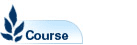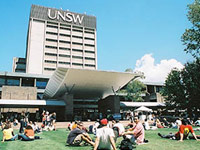# Mathematics 1A - MATH1131

Faculty: Faculty of Science

Course Outline: http://www.maths.unsw.edu.au/

Campus: Sydney

Units of Credit: 6

Indicative Contact Hours per Week: 6

Enrolment Requirements:

Exclusion: ECON1202, ECON2291, MATH1031, MATH1141, MATH1151

Excluded: ECON1202, ECON2291, MATH1031, MATH1141, MATH1151

Tuition Fee: See Tuition Fee Schedule

Further Information: See Class Timetable

View course information for previous years.

## Description

Complex numbers, vectors and vector geometry, linear equations, matrices and matrix algebra, determinants. Functions, limits, continuity and differentiability, integration, polar coordinates, logarithms and exponentials, hyperbolic functions. Introduction to computing and the Maple symbolic algebra package.

Assumed knowledge: HSC Mathematics Extension 1. Students will be expected to have achieved a combined mark of at least 100 in Mathematics and Mathematics Extension 1.